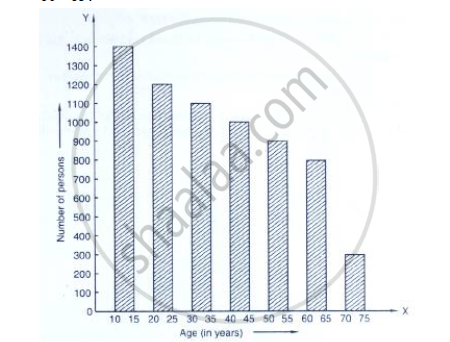# Study the Bar Graph Representing the Number of Persons in Various Age Groups in a Town Shown in Fig. Below. Observe the Bar Graph and Answer the Following Questions: - Mathematics

Study the bar graph representing the number of persons in various age groups in a town shown in Fig. below. Observe the bar graph and answer the following questions:
(i) What is the percentage of the youngest age-group persons over those in the oldest age group?
(ii) What is the total population of the town?

(iii) What is the number of persons in the age group 60 - 65?
(iv) How many persons are more in the age-group 10 - 15 than in the age group 30 - 35?
(v) What is the age-group of exactly 1200 persons living in the town?
(vi) What is the total number of persons living in the town in the age-group 50 - 55?
(vii) What is the total number of persons living in the town in the age-groups 10 - 15 and 60 - 65?(viii) Whether the population in general increases, decreases or remains constant with the increase in the age-group.

#### Solution

(i) The percentage of the youngest age group persons over those in the oldest age group
= 1400/300 × 100

= 466 2/3
(ii) Total population of the town
=1400 +1200+1100+1000+900+800+300

=6700.
(iii) The number of persons in the age group of 60- 65 is 800.
(iv) The number of persons are more in the age group 10-15 than in the age group
=30-35 =1400-1100 = 300.
(v) The age group in which exactly 1200 persons living in the town is 20-25
(vi) The total number of persons living in the town in the age-group 50-55 is 900.
(vii) The total number of persons living in the town in the age group 10 to 15 and
60-65 =1400+800
= 2,200
(viii) The population decreases with the increase in the group.

Concept: Graphical Representation of Data
Is there an error in this question or solution?

#### APPEARS IN

RD Sharma Mathematics for Class 9
Chapter 23 Graphical Representation of Statistical Data
Exercise 23.1 | Q 5 | Page 8

Share